• 基于Visual Studio的Opencv进行图像的仿射变换，通过构建仿射矩阵来旋转图像。图像处理
• 因此，几何变换的功能，在现实生活里的需求必不可少。 为了理解这个几何的问题，可以来回忆一下初中的课本内容： 从这里可以看到平移的基本性质，有了这些概念之后，就要进入解释几何，平移的表达，比如往x轴移动...
• 仿射变换的两种方式： 1、利用变换前后的三个坐标点达到变换目的。 2、使用旋转角度和缩放倍数来变换。 在实际的使用中，采用1的方式比较方便，但是有一个问题：变换之后的矩阵大小该如何正确设置，如果设置...
仿射变换的两种方式：
1、利用变换前后的三个坐标点达到变换目的。
2、使用旋转角度和缩放倍数来变换。

在实际的使用中，采用1的方式比较方便，但是有一个问题：变换之后的矩阵大小该如何正确设置，如果设置大小不合适，会导致图的实际区域超出边界。所以在此记录一下解决办法。

思路：由于已知了变换前后的三个坐标点，可以先求得变换的旋转角度，缩放倍数则看实际的项目需求。之后使用方法2来求个仿射变换矩阵。
//求得旋转角度

cv::Point po1;//变换前坐标点1
cv::Point po2;//变换前坐标点2
cv::Point po3;//变换后坐标点1
cv::Point po4;//变换后坐标点2

int x1 = po2.x - po1.x;
int y1 = po2.y - po1.y;
int x2 = po4.x - po3.x;
int y2 = po4.y - po3.y;
double d1 = atan2((double)x1, (double)y1) * (180.0 / CV_PI);
double d2 = atan2((double)x2, (double)y2) * (180.0 / CV_PI);
double angle = d1 - d2;
if (angle < 0.0)
{
angle += 360.0;
}

//开始变换
cv::Point2f center(image.cols / 2, image.rows / 2);
double scale = 1.0;
cv::Mat warp = getRotationMatrix2D(center, angle, 1.0);

//找出变换之后图像的实际区域
int rotated_width = ceil(image.rows * fabs(sin(angle * CV_PI / 180)) + image.cols * fabs(cos(angle * CV_PI / 180)));
int rotated_height = ceil(image.cols * fabs(sin(angle * CV_PI / 180)) + image.rows * fabs(cos(angle * CV_PI / 180)));
//修改变换中心
warp.at<double>(0, 2) += (rotated_width - image.cols) / 2;
warp.at<double>(1, 2) += (rotated_height - image.rows) / 2;

cv::Mat dstImage;
warpAffine(image, dstImage, warp, cv::Size(rotated_width,rotated_height));
cv::Point top, buttom, left, right;
//搜边取出实际区域
for (int i = 0; i < dstImage.rows; i++)
{
int flag = 0;
for (int j = 0; j < dstImage.cols; ++j)
{
cv::Vec3b rgb = dstImage.at<cv::Vec3b>(i, j);
if (rgb > 0 || rgb > 0 || rgb > 0)
{
top.x = j; top.y = i;
flag = 1;
break;
}
}
if (flag == 1)
break;
}
for (int i = dstImage.rows - 1; i >= 0; i--)
{
int flag = 0;
for (int j = 0; j < dstImage.cols; j++)
{
cv::Vec3b rgb = dstImage.at<cv::Vec3b>(i, j);
if (rgb > 0 || rgb > 0 || rgb > 0)
{
buttom.x = j; buttom.y = i;
flag = 1;
break;
}
}
if (flag == 1)
break;
}
for (int i = 0; i < dstImage.cols; i++)
{
int flag = 0;
for (int j = 0; j < dstImage.rows; j++)
{
cv::Vec3b rgb = dstImage.at<cv::Vec3b>(j, i);
if (rgb > 0 || rgb > 0 || rgb > 0)
{
left.x = i; left.y = j;
flag = 1;
break;
}
}
if (flag == 1)
break;
}
for (int i = dstImage.cols - 1; i >= 0; i--)
{
int flag = 0;
for (int j = 0; j < dstImage.rows; j++)
{
cv::Vec3b rgb = dstImage.at<cv::Vec3b>(j, i);
if (rgb > 0 || rgb > 0 || rgb > 0)
{
right.x = i; right.y = j;
flag = 1;
break;
}
}
if (flag == 1)
break;
}

cv::Rect rect;
rect.x = left.x;
rect.y = top.y;
rect.width = right.x - left.x;
rect.height = buttom.y - top.y;
cv::Mat del = dstImage(rect);

//del即为变换之后图像的实际区域(去除掉黑边)

展开全文• def get_affine_matrix(v1, v2, v3, new_v1, new_v2, new_v3): ... 根据三个坐标点的坐标变换获取相对应仿射矩阵 """ pts = np.float32([[v1, v1], [v2, v2], [v3, v3]]) pts1 = np.float32([[...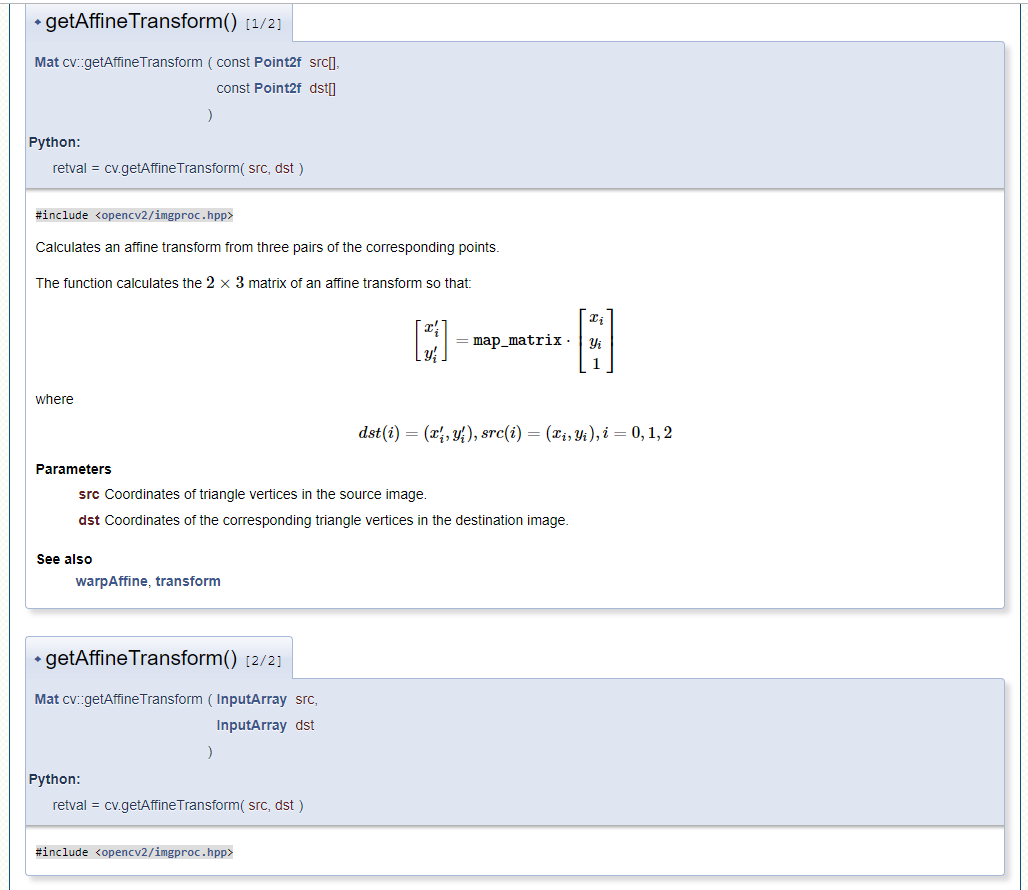def get_affine_matrix(v1, v2, v3, new_v1, new_v2, new_v3):
"""
根据三个坐标点的坐标变换获取相对应仿射矩阵
"""
pts = np.float32([[v1, v1], [v2, v2], [v3, v3]])
pts1 = np.float32([[new_v1, new_v1], [new_v2, new_v2], [new_v3, new_v3]])
affine_matrix = cv2.getAffineTransform(pts, pts1)
return affine_matrix

展开全文• 在前面实现了平移和缩放，还有一种常用的坐标变换，那就是旋转。比如拍摄的照片上传到电脑里，再打开时发现人的头在下面脚在上，这样肯定看不了，那么就需要把这个照片旋转180度才可以接受。由于旋转变换，都是绕着...
• 仿射变换原理 仿射变换（Affine Transformation或 Affine Map），又称仿射映射，是指在几何中，一个向量空间进行一次线性变换并接上一个平移，变换为另一个向量空间的过程。它保持了二维图形的“平直性”（即：直线...
目录
仿射变换原理
opencv中仿射变换实现
使用getAffineTransform()函数求仿射变换矩阵
使用 getRotationMatrix2D()函数获取仿射矩阵
使用仿射矩阵对图像做仿射变换warpAffine

仿射变换原理
仿射变换（Affine Transformation或 Affine Map），又称仿射映射，是指在几何中，一个向量空间进行一次线性变换并接上一个平移，变换为另一个向量空间的过程。它保持了二维图形的“平直性”（即：直线经过变换之后依然是直线）和“平行性”（即：二维图形之间的相对位置关系保持不变，平行线依然是平行线，且直线上点的位置顺序不变）。
一个任意的仿射变换都能表示为乘以一个矩阵(线性变换)接着再加上一个向量(平移)的形式。可以表示为线性变换和平移变换的叠加
我们能够用仿射变换来表示如下三种常见的变换形式：
旋转，rotation (线性变换)平移，translation(向量加)缩放，scale(线性变换)
如果进行更深层次的理解，仿射变换代表的是两幅图之间的一种映射关系。
仿射变换的数学表示是先乘以一个线形变换矩阵再加上一个平移向量，其中线性变换矩阵为2×2的矩阵，平移向量为2×1的向量
假设我们存在一个线性变换矩阵 A 和平移矩阵B  ，两者与输入的M矩阵之间的关系如式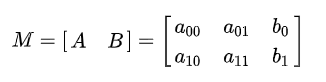根据旋转矩阵 A和平移矩阵B 以及图像像素值，仿射变换的数学原理可以用式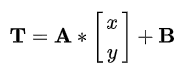仿射变换又称为三点变换，如果知道变换前后两张图像中三个像素点坐标的对应关系，就可以求得仿射变换中的变换矩阵 .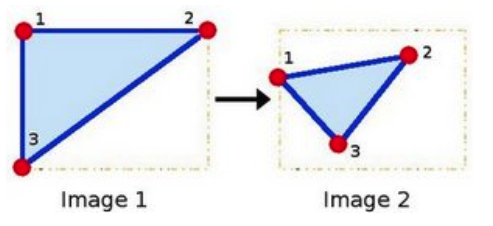点1, 2 和 3 (在图一中形成一个三角形) 与图二中三个点一一映射, 仍然形成三角形, 但形状已经大大改变. 如果我们能通过这样两组三点求出仿射变换 (你能选择自己喜欢的点), 接下来我们就能把仿射变换应用到图像中所有的点.。
而我们通常使用2 x 3的矩阵来表示仿射变换。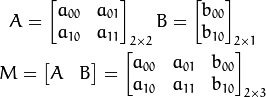opencv中仿射变换实现
要求出图像的仿射变换（旋转缩放等），首先要求出仿射变换矩阵。
使用getAffineTransform()函数求仿射变换矩阵
上文提到过，已知变换前后两张图像中三个像素点坐标的对应关系，就可以求得仿射变换中的变换矩阵。OpenCV 4提供了利用三个对应像素点来确定 矩阵的函数getAffineTransform()
函数原型：
CV_EXPORTS Mat getAffineTransform( const Point2f src[], const Point2f dst[] );

Calculates an affine transform from three pairs of the corresponding points. The function calculates the  matrix of an affine transform so that:

where

@param src Coordinates of triangle vertices in the source image. @param dst Coordinates of the corresponding triangle vertices in the destination image.
src[]：原图像中的三个像素坐标。dst[]：目标图像中的三个像素坐标。

使用 getRotationMatrix2D()函数获取仿射矩阵
函数原型：
CV_EXPORTS_W Mat getRotationMatrix2D(Point2f center, double angle, double scale);

/** @sa getRotationMatrix2D */
CV_EXPORTS Matx23d getRotationMatrix2D_(Point2f center, double angle, double scale);

inline
Mat getRotationMatrix2D(Point2f center, double angle, double scale)
{
return Mat(getRotationMatrix2D_(center, angle, scale), true);
}

@brief Calculates an affine matrix of 2D rotation.
The function calculates the following matrix:以此得出仿射矩阵：

where

The transformation maps the rotation center to itself. If this is not the target, adjust the shift.
@param center Center of the rotation in the source image. @param angle Rotation angle in degrees. Positive values mean counter-clockwise rotation (the coordinate origin is assumed to be the top-left corner). @param scale Isotropic scale factor.
第一个参数，Point2f类型的center，表示源图像的旋转中心。第二个参数，double类型的angle，旋转角度。角度为正值表示向逆时针旋转（坐标原点是左上角）。第三个参数，double类型的scale，缩放系数。

getRotationMatrix2D函数源代码：
cv::Mat cv::getRotationMatrix2D( Point2f center,double angle, double scale )
{
angle *= CV_PI/180;
double alpha = cos(angle)*scale;
double beta = sin(angle)*scale;

Mat M(2, 3, CV_64F);//生成一个2*3的矩阵
double* m = (double*)M.data;

//按照上文公式填充内容
m = alpha;
m = beta;
m = (1-alpha)*center.x - beta*center.y;
m = -beta;
m = alpha;
m = beta*center.x + (1-alpha)*center.y;

return M;
}

使用仿射矩阵对图像做仿射变换warpAffine
warpAffine函数原型：
CV_EXPORTS_W void warpAffine( InputArray src, OutputArray dst,
InputArray M, Size dsize,
int flags = INTER_LINEAR,
int borderMode = BORDER_CONSTANT,
const Scalar& borderValue = Scalar());

@brief Applies an affine transformation to an image.
The function warpAffine transforms the source image using the specified matrix:依据如下式子，对图像做仿射变换

when the flag #WARP_INVERSE_MAP is set. Otherwise, the transformation is first inverted with #invertAffineTransform and then put in the formula above instead of M. The function cannot operate in-place.
@param src input image. @param dst output image that has the size dsize and the same type as src . @param M  transformation matrix. @param dsize size of the output image. @param flags combination of interpolation methods (see #InterpolationFlags) and the optional flag #WARP_INVERSE_MAP that means that M is the inverse transformation (). @param borderMode pixel extrapolation method (see #BorderTypes); when borderMode=#BORDER_TRANSPARENT, it means that the pixels in the destination image corresponding to the "outliers" in the source image are not modified by the function. @param borderValue value used in case of a constant border; by default, it is 0.
第一个参数，InputArray类型的src，输入图像，即源图像，填Mat类的对象即可。第二个参数，OutputArray类型的dst，函数调用后的运算结果存在这里，需和源图片有一样的尺寸和类型。第三个参数，InputArray类型的M，2×3的变换矩阵。第四个参数，Size类型的dsize，表示输出图像的尺寸。第五个参数，int类型的flags，插值方法的标识符。此参数有默认值INTER_LINEAR(线性插值)，可选的插值方式如下：

INTER_NEAREST - 最近邻插值INTER_LINEAR - 线性插值（默认值）INTER_AREA - 区域插值INTER_CUBIC –三次样条插值INTER_LANCZOS4 -Lanczos插值CV_WARP_FILL_OUTLIERS - 填充所有输出图像的象素。如果部分象素落在输入图像的边界外，那么它们的值设定为 fillval.CV_WARP_INVERSE_MAP –表示M为输出图像到输入图像的反变换，即 。因此可以直接用来做象素插值。否则, warpAffine函数从M矩阵得到反变换。
第六个参数，int类型的borderMode，边界像素模式，默认值为BORDER_CONSTANT。第七个参数，const Scalar&类型的borderValue，在恒定的边界情况下取的值，默认值为Scalar()，即0。

使用两种方式进行仿射变换：
#include <opencv2\opencv.hpp>
#include <iostream>
#include <vector>

using namespace std;
using namespace cv;

int main()
{
if (img.empty())
{
cout << "请确认图像文件名称是否正确" << endl;
return -1;
}

Mat rotation0, rotation1, img_warp0, img_warp1;
double angle = 30;  //设置图像旋转的角度
Size dst_size(img.rows, img.cols);  //设置输出图像的尺寸
Point2f center(img.rows / 2.0, img.cols / 2.0);  //设置图像的旋转中心
rotation0 = getRotationMatrix2D(center, angle, 1);  //计算放射变换矩阵
warpAffine(img, img_warp0, rotation0, dst_size);  //进行仿射变换
imshow("img_warp0", img_warp0);
//根据定义的三个点进行仿射变换
Point2f src_points;
Point2f dst_points;
src_points = Point2f(0, 0);  //原始图像中的三个点
src_points = Point2f(0, (float)(img.cols - 1));
src_points = Point2f((float)(img.rows - 1), (float)(img.cols - 1));
//放射变换后图像中的三个点
dst_points = Point2f((float)(img.rows)*0.11, (float)(img.cols)*0.20);
dst_points = Point2f((float)(img.rows)*0.15, (float)(img.cols)*0.70);
dst_points = Point2f((float)(img.rows)*0.81, (float)(img.cols)*0.85);
rotation1 = getAffineTransform(src_points, dst_points);  //根据对应点求取仿射变换矩阵
warpAffine(img, img_warp1, rotation1, dst_size);  //进行仿射变换
imshow("img_warp1", img_warp1);
waitKey(0);
return 0;
}

实验效果：参考文章：
【从零学习OpenCV 4】图像仿射变换
【OpenCV入门教程之十八】OpenCV仿射变换 & SURF特征点描述合辑
展开全文计算机视觉
• 图像img的像素为534*300，img.shape = (300, 534, 3)。 需要引入： import cv2 as cv import numpy as np ...img.shape得到的(300, 534, 3)，前者300是高度，y轴的值...cv2.warpAffine() 仿射变换（从二维坐标到二维
图像img的像素为534*300，img.shape = (300, 534, 3)。需要引入：
import cv2 as cv
import numpy as np
img.shape得到的(300, 534, 3)，前者300是高度，y轴的值，后者534是宽度，x轴的值，这一点有些不同。
图像的xy轴，是以图像左上角顶点为(0, 0)原点，类似于css。从顶点沿宽度向右是x正轴，沿高度向下是y正轴。
一、平移变换

cv2.warpAffine()   仿射变换（从二维坐标到二维坐标之间的线性变换，且保持二维图形的“平直性”和“平行性”。仿射变换可以通过一系列的原子变换的复合来实现，包括平移，缩放，翻转，旋转和剪切）
参数：
img: 图像对象
M：2*3 transformation matrix (转变矩阵)
dsize：输出矩阵的大小,注意格式为（cols，rows）  即width对应cols，height对应rows
flags：可选，插值算法标识符，有默认值INTER_LINEAR，
如果插值算法为WARP_INVERSE_MAP, warpAffine函数使用如下矩阵进行图像转dst(x,y)=src(M11*x+M12*y+M13,M21*x+M22*y+M23)
borderMode：可选， 边界像素模式，有默认值BORDER_CONSTANT
borderValue:可选，边界取值，有默认值Scalar()即0

下面的M矩阵表示向x轴正方向（向右）移动200像素，向y轴负方向（向上）移动100像素。
# 创建转变矩阵
M = np.float32([[1, 0, 200], [0, 1, -100]])
# 使用warpAffine()方法执行变换
img = cv.warpAffine(img, M, (500, 300))
# 展示图片
cv.imshow("img", img)
cv.waitKey(0)二、缩放变换
缩放变换的转变矩阵与平移变换的转变矩阵是一样的。
M是一个2*3矩阵，[[1, 0, 100], [0, 1, 200]]。
从左到右看，第一个“1”代表x轴（长度）是原来的几倍，“100”表示将原图沿x轴如何移动。
第二个“1”表示y轴（宽度）是原来的几倍，“100”表示将原图沿y轴如何移动。
下面的操作表示将原图放大1.5倍。

# 创建转变矩阵
M = np.float32([[1.5, 0, 0], [0, 1.5, 0]])
# 执行变换，输出图片大小为500*400
img = cv.warpAffine(img, M, (500, 400))
# 展示图片
cv.imshow("img", img)
cv.waitKey(0)三、旋转变换
通过getRotationMatrix2D()能得到转变矩阵M。

　cv2.getRotationMatrix2D()  返回2*3的转变矩阵（浮点型）
参数：
center：旋转的中心点坐标
angle：旋转角度，单位为度数，证书表示逆时针旋转
scale：同方向的放大倍数

我们以图片的左上角（0，0）原点为旋转中心，旋转30°。
# 创建旋转转变矩阵
M = cv.getRotationMatrix2D((0, 0), 30, 1)
# 执行转变
img = cv.warpAffine(img, M, (500, 300))

cv.imshow("img", img)
cv.waitKey(0)四、三点定位，仿射变换矩阵的计算，cv2.getAffineTransform()
通过图片变换前后的三组坐标定位，和cv2.getAffineTransform()方法，可以计算出我们需要的仿射变换矩阵M。
该方法可以一定程度上代替上面三个方法，同时实现旋转、平移和缩放。
getAffineTransform()等同于将平移，旋转和缩放的变换矩阵相乘，最后都会获得仿射变换矩阵。

cv2.getAffineTransform()  返回2*3的转变矩阵
参数：
src：原图像中的三组坐标，如np.float32([[50,50],[200,50],[50,200]])
dst: 转换后的对应三组坐标，如np.float32([[10,100],[200,50],[100,250]])

img原图的四个角的坐标为[0, 0] , [534, 0], [0,300], [534, 300]。（此处为[x, y] 坐标形式，不同于shape）
# 原图像中的三组坐标
pts1 = np.float32([[0, 0] , [534, 0], [534, 300]])
# 转换后的三组对应坐标
pts2 = np.float32([[300, 0], [300, 534], [0, 534]])
# 计算仿射变换矩阵
M = cv.getAffineTransform(pts1, pts2)
# 执行变换
img = cv.warpAffine(img, M ,(300, 534))二维仿射变换总结：我们可以发现，上面的所有仿射变换矩阵M都是一个2*3的矩阵，而用来进行变换的方法都是同一个cv2.warpAffine()。
下面是三维仿射变换
五、透视变换（三维）
与四、三点定位，仿射变换矩阵的计算类似，三维的仿射变换矩阵需要四组坐标来进行定位。
与之前不同的是，我们需要使用另外两个方法getPerspectiveTransform()和warpPerspective()，仿射变换矩阵M变成了3*3矩阵。

cv2.getPerspectiveTransform()   返回3*3的转变矩阵
参数：
src：原图像中的四组坐标，如 np.float32([[56,65],[368,52],[28,387],[389,390]])
dst: 转换后的对应四组坐标，如np.float32([[0,0],[300,0],[0,300],[300,300]])

cv2.warpPerspective()
参数：
src: 图像对象
M：3*3 transformation matrix (转变矩阵)
dsize：输出矩阵的大小，注意格式为（cols，rows）  即width对应cols，height对应rows
flags：可选，插值算法标识符，有默认值INTER_LINEAR，
如果插值算法为WARP_INVERSE_MAP, warpAffine函数使用如下矩阵进行图像转dst(x,y)=src(M11*x+M12*y+M13,M21*x+M22*y+M23)
borderMode：可选， 边界像素模式，有默认值BORDER_CONSTANT
borderValue:可选，边界取值，有默认值Scalar()即0

我们将img图片的左上角和右上角往“里”缩一缩，同时左下角和右下角位置不变。
# 原图的四组顶点坐标
pts3D1 = np.float32([[0, 0], [534, 0], [0, 300], [534, 300]])
# 转换后的四组坐标
pts3D2 = np.float32([[100, 100], [434, 100], [0, 300], [534, 300]])
# 计算透视放射矩阵
M = cv.getPerspectiveTransform(pts3D1, pts3D2)
# 执行变换
img = cv.warpPerspective(img, M, (550， 400))展开全文python 计算机视觉 图像处理
• 本部分介绍了OpenCV-Python的getAffineTransform函数，并通过在图像上任选三个点指定为结果图像的左上角、左下角、右下角，从而实现原图像三个点圈定范围的子图像通过仿射变换实现整体放大。图形图像处理 编程语言 程序开发
• OpenCV仿射变换——旋转一、原理与数学推导1.1图示1.2公式推导：二、实现代码三、运行结果3.1图片效果：3.2仿射矩阵M 一、原理与数学推导 1.1图示 1、旋转前和旋转后位置关系图，θ是旋转的角度 2、首先需要平移一...
• 仿射变换中，原图中所有的平行线在结果图像中同样平行。为了创建这个矩阵需要从原图像中找到三个点以及他们在输出图像中的位置。然后cv2.getAﬃneTransform 会创建一个 2x3 的矩阵，最后这个矩阵会被传给函数 cv2....
• 1、C++仿射变换中主要涉及到2个函数：getAffineTransform() 和warpAffine() 2、粗略的理解：getAffineTransform()是用来求得仿射变换矩阵的；而warpAffine()是用来对原图像进行仿射变换的，从而得到目标图像。 3、...
• OpenCV仿射变换——平移 公式及原理 实现代码 #include<iostream> #include<opencv2/core.hpp> #include<opencv2/imgproc.hpp> #include<opencv2/highgui.hpp> int main() { cv::Mat src ...
• /******************************方式一******************************/ //第一种仿射变换的调用方式：三点法 Point[] srcPoints = new Point; //原图中的三点 Point[] dstPoints = new Point; //目标图中的...
• 仿射变换 1) 用途 旋转 (线性变换)，平移 (向量加)．缩放(线性变换)，错切，反转 2) 方法 仿射变换是一种二维坐标到二维坐标之间的线性变换，它保持了二维图形的“平直性”（直线经过变换之后依然是直线）和“平行...
• 常见的2D图像变换从原理上讲主要包括基于2×3矩阵的仿射变换和基于3×3矩阵透视变换。 仿射变换 原理 基本的图像变换就是二维坐标的变换：从一种二维坐标(x,y)到另一种二维坐标(u,v)的线性变换： 如果写成矩阵的...
• 这样的需求，就需要使用仿射变换的缩放功能，而实现这个功能的方法，就是采用齐次坐标的变换功式： 可看到最后一条公式，就是缩放公式，要实现二维图像的缩放，需要构造前面那个缩放矩阵，因此在OpenCV也是构造一个...
• OpenCV仿射变换+投射变换+单应性矩阵 estimateRigidTransform()：计算多个二维点对或者图像之间的最优仿射变换矩阵 （2行x3列），H可以是部分自由度，比如各向一致的切变。 getAffineTransform()：计算3个二维点对...
• import cv2 import numpy as np from matplotlib import pyplot as plt img = cv2.imread('pic3.PNG') cv2.imshow('img', img) cv2.waitKey(0) cv2.destroyAllWindows() rows, cols = img.shape[:2] ...
• 仿射变换原理 仿射变换是线性变换，有一张图可以很好地展示放射变换的效果 其实仿射变换是透视变换的一种特例，但是透视变换的自由度更高，3*3的矩阵表示，透视变换的自由度是8，而放射变换可以用2*3的矩阵表示，...
• // ConsoleApplication1.cpp : 定义控制台应用程序的入口点。 ...opencv2\opencv.hpp> #include<opencv2\highgui\highgui.hpp> #include<vector> using namespace cv; using nam...
• 一、话说仿射变换和透视变换对于平面区域，有两种方式的几何转换：一种是基于2×3矩阵进行的变换，叫仿射变换；另一种是基于3×3矩阵进行的变换，叫透视变换或者单应性映射。关于仿射变换和透射变换的矩阵变换，这篇...
• OpenCV中，目前并没有现成的函数直接用来实现图像旋转，它是用仿射变换函数cv::warpAffine来实现的，此函数目前支持4种插值算法，最近邻、双线性、双三次、兰索斯插值，如果传进去的参数为基于像素区域关系插值...
• 本篇文章中，我们一起探讨了OpenCV仿射变换和SURF特征点描述相关的知识点，主要一起了解OpenCV仿射变换相关的函数warpAffine和getRotationMatrix2D，SURF算法在OpenCV中进一步的体现与应用。此博文一共有两个...c++ 图像处理 SURF
• 1图像转换 OpenCV提供了两个转换函数cv2.warpAffine和cv2.warpPerspective，可以使用它们...cv2.warpAffine采用2x3变换矩阵，而cv2.warpPerspective采用3x3变换矩阵作为输入。 2图像缩放 缩放只是调整图像大...
• 仿射变换 仿射变换，是指在几何中，一个向量空间进行一次线型变换并接上一个平移，变换为另一个向量空间的过程。它保持了二维图形的“平直性”（直线经过变换之后仍然是直线）和“平行性”（直线经过变换之后仍然是...计算机视觉 c++...